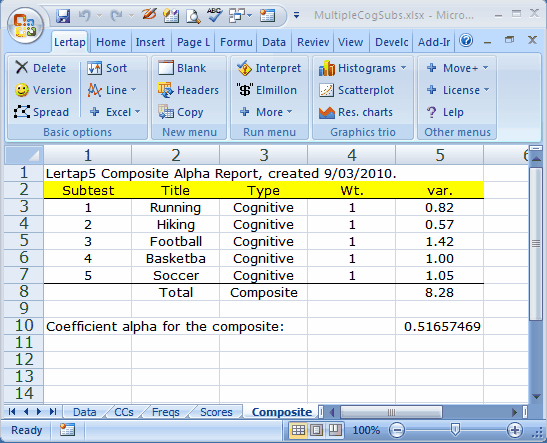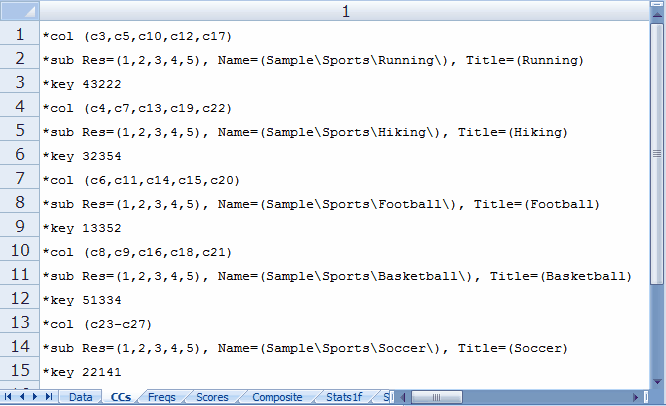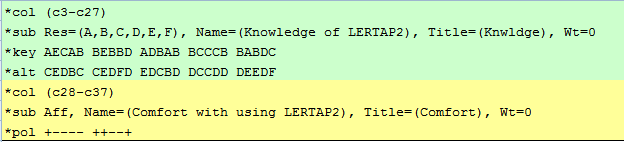﻿ TotalTest1

# TotalTest1

The TotalTest1 macro produces output exemplified here when running Lertap 5 with Excel 2007:The CCs lines corresponding to this output are shown here:This example involves five cognitive subtests.

The information displayed in the Composite Alpha Report is derived from various sources. The Titles are taken from the CCs lines, as are the values seen in the Wt. column (a subtest's Wt. value will equal 1 (one) unless Wt= assignments are made on the *sub line). "var." values are taken from the Scores worksheet.

The Total score is a composite:

Total = Sum ( Wt(j) * X(j) )

where Wt(j) is the 'weight' assigned to subtest 'j', and X(j) is a student's score on subtest 'j'. The Sum is taken over all J subtests whose Wt. is not equal to 0 (zero).

Let V(j) be the variance (var.) of subtest j, and V(T) the variance of the Total score.

Then

Let P1 = Sum ( Wt(j)2 * V(j) )

Let P2 = J / (J-1)

Let P3 = P1 / V(T)

then

alpha = P2 * ( 1 - P3 )

In this example, alpha = 0.51657469

To keep a subtest from entering the Total score, set Wt=0 on the subtest's *sub line.In this example, all subtests (J=2) have Wt=0, and no Total score will be made.

The TotalTest1 macro will only work when two or more subtests have non-zero Wt. values. The subtests do not have to be of the same type, that is, some may be cognitive while others are affective.

(This macro was created at the request of a veteran Lertap user who knew that composite alpha used to be computed by the mainframe version of Lertap, Lertap 2. The P1, P2, and P3 terms are as seen on pp. 278 - 281 of the Lertap 2 user guide (Nelson, 1974).)